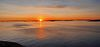# Patrik Jansson

More### Patrik Jansson

Professor of Computer Science

+46317725415

# Level-p-complexity of Boolean Functions (an update)

March 12, 2023

In an earlier post (2022-12) I mentioned work in progress and this has now (2023-02) lead to a submission of "Level-p-complexity of Boolean functions using Thinning, Memoization, and Polynomials" to the Journal of Functional Programming. A preprint is available as arXiv:2302.02473, the Haskell source code is on GitHub. I gave a talk 2023-03 as part of the Chalmers FP seminar series and the recording is available on YouTube (and slides here).

Abstract:
This paper describes a purely functional library for computing level-p-complexity of Boolean functions, and applies it to two-level iterated majority. Boolean functions are simply functions from n bits to one bit, and they can describe digital circuits, voting systems, etc. An example of a Boolean function is majority, which returns the value that has majority among the n input bits for odd n. The complexity of a Boolean function f measures the cost of evaluating it: how many bits of the input are needed to be certain about the result of f. There are many competing complexity measures but we focus on level-p-complexity -- a function of the probability p that a bit is 1. The level-p-complexity $$D_p(f)$$ is the minimum expected cost when the input bits are independent and identically distributed with Bernoulli(p) distribution. We specify the problem as choosing the minimum expected cost of all possible decision trees -- which directly translates to a clearly correct, but very inefficient implementation. The library uses thinning and memoization for efficiency and type classes for separation of concerns. The complexity is represented using polynomials, and the order relation used for thinning is implemented using polynomial factorisation and root-counting. Finally we compute the complexity for two-level iterated majority and improve on an earlier result by J. Jansson.

Share